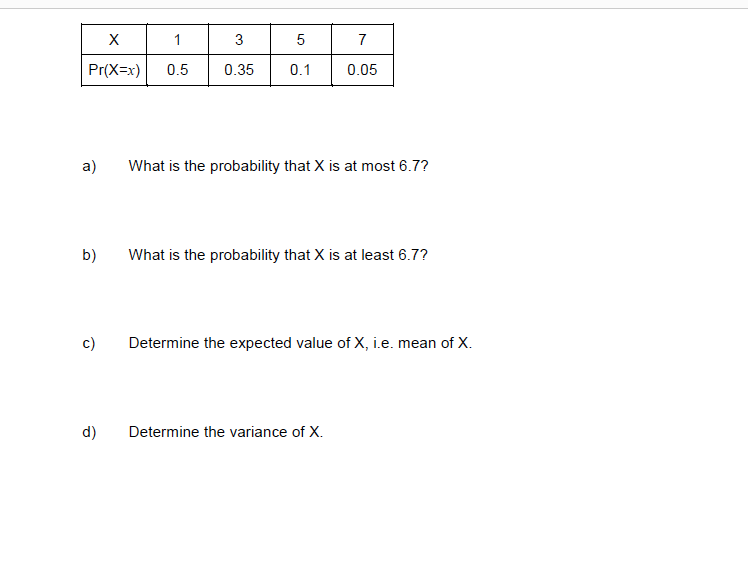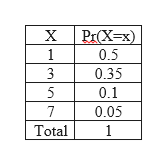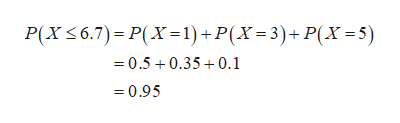X157Pr(X x)0.50.350.10.05a)What is the probability that X is at most 6.7?b)What is the probability that X is at least 6.7?Determine the expected value of X, i.e. mean of Xc)Determine the variance of X.d)

Questionhelp_outlineImage TranscriptioncloseX 1 5 7 Pr(X x) 0.5 0.35 0.1 0.05 a) What is the probability that X is at most 6.7? b) What is the probability that X is at least 6.7? Determine the expected value of X, i.e. mean of X c) Determine the variance of X. d) fullscreen
Step 1

The given probability distribution is shown below:help_outlineImage TranscriptioncloseX Р(Х-х) 1 0.5 3 0.35 5 0.1 7 0.05 Total 1 fullscreen
Step 2

a.

The probability that X is at most 6.7 is obtained as follows:help_outlineImage TranscriptioncloseP(XS6.7) P(X-1) + P(X=3)+ P(X=5) 0.50.350.1 =0.95 fullscreen
Step 3

b.

The probability that X is at least 6.7 is ob...

Want to see the full answer?

See Solution

Want to see this answer and more?

Our solutions are written by experts, many with advanced degrees, and available 24/7

See Solution
Tagged in

Statistics